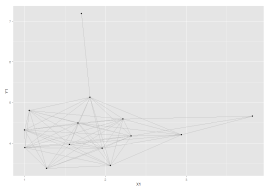# Getting ggplot2 to work with igraph

Published on
7 Dec 2015
Written by
Jonathan Bright

One common criticism of the otherwise excellent ggplot2 is that it doesn’t come with network visualisation capability. Network vis is so popular at the moment that it seems like a bit of a big omission; but network data is also quite unique in terms of structure (and the layout algorithms would need implementing) so I can see why it hasn’t been integrated.

Moritz Marbach has a great post explaining how to easily get ggplot2 up and running with network data. It was still one of the top hits on Google when I checked it out recently for a project. However the post is from 2011 so is getting a little dated – it uses the sna package rather than igraph (which seems to be becoming a standard for network science) and also has a few deprecated ggplot2 commands in it. So I thought I’d add a bit of an update here to the code.

As Marbach explains the secret to getting ggplot2 to draw networks is quite simple: get a network analysis package to give you a list of nodes, edges, and node layout information as a series of X,Y coordinates. Then you can simply plot the nodes with `geom_point` and the edges with `geom_segment`. Put together it looks something like this:

`library(igraph)`

`g = read.graph("a-network.gml", format="gml")`

`#get the node coordinates`
```plotcord <- data.frame(layout.fruchterman.reingold(g)) colnames(plotcord) = c("X1","X2")```

```#get edges, which are pairs of node IDs edgelist <- get.edgelist(g)```

```#convert to a four column edge data frame with source and destination coordinates edges <- data.frame(plotcord[edgelist[,1],], plotcord[edgelist[,2],]) colnames(edges) <- c("X1","Y1","X2","Y2")```

`ggplot() + geom_segment(aes(x=X1, y=Y1, xend = X2, yend = Y2), data=edges, size = 0.5, colour="grey") + geom_point(aes(X1, X2), data=plotcord)`

Output:OK it still needs some work! But anyone familiar with ggplot2 can do the rest.